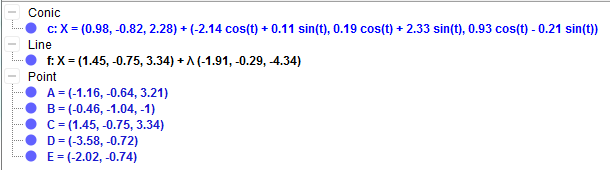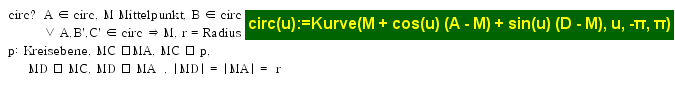# paramertic equation for circle in 3d?

alirezagholamian shared this question 1 year ago

Hi

how Geogebra calculate parametric equation of circle in 3d?

thanks1

W.F.1

this is a tool, I want parametric curve of circle such as c in your file.1

How geogebra find parametric curve c?1

What sort of answer do you want? You can see exactly how it does it if you know Java https://github.com/geogebra...1

I do not know Java very well

I want matematical metod1

oder dieses?

W.F.1

If possible, write down other methods you know.